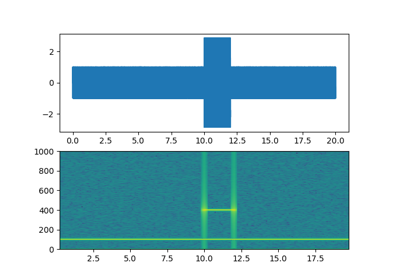matplotlib.pyplot.specgram¶

matplotlib.pyplot.specgram(x, NFFT=None, Fs=None, Fc=None, detrend=None, window=None, noverlap=None, cmap=None, xextent=None, pad_to=None, sides=None, scale_by_freq=None, mode=None, scale=None, vmin=None, vmax=None, *, data=None, **kwargs)[source]

Plot a spectrogram.

Compute and plot a spectrogram of data in x. Data are split into NFFT length segments and the spectrum of each section is computed. The windowing function window is applied to each segment, and the amount of overlap of each segment is specified with noverlap. The spectrogram is plotted as a colormap (using imshow).

Parameters: x : 1-D array or sequence Array or sequence containing the data. Fs : scalar The sampling frequency (samples per time unit). It is used to calculate the Fourier frequencies, freqs, in cycles per time unit. The default value is 2. window : callable or ndarray A function or a vector of length NFFT. To create window vectors see window_hanning, window_none, numpy.blackman, numpy.hamming, numpy.bartlett, scipy.signal, scipy.signal.get_window, etc. The default is window_hanning. If a function is passed as the argument, it must take a data segment as an argument and return the windowed version of the segment. sides : {'default', 'onesided', 'twosided'} Specifies which sides of the spectrum to return. Default gives the default behavior, which returns one-sided for real data and both for complex data. 'onesided' forces the return of a one-sided spectrum, while 'twosided' forces two-sided. pad_to : int The number of points to which the data segment is padded when performing the FFT. This can be different from NFFT, which specifies the number of data points used. While not increasing the actual resolution of the spectrum (the minimum distance between resolvable peaks), this can give more points in the plot, allowing for more detail. This corresponds to the n parameter in the call to fft(). The default is None, which sets pad_to equal to NFFT NFFT : int The number of data points used in each block for the FFT. A power 2 is most efficient. The default value is 256. This should NOT be used to get zero padding, or the scaling of the result will be incorrect. Use pad_to for this instead. detrend : {'none', 'mean', 'linear'} or callable, default 'none' The function applied to each segment before fft-ing, designed to remove the mean or linear trend. Unlike in MATLAB, where the detrend parameter is a vector, in Matplotlib is it a function. The mlab module defines detrend_none, detrend_mean, and detrend_linear, but you can use a custom function as well. You can also use a string to choose one of the functions: 'none' calls detrend_none. 'mean' calls detrend_mean. 'linear' calls detrend_linear. scale_by_freq : bool, optional Specifies whether the resulting density values should be scaled by the scaling frequency, which gives density in units of Hz^-1. This allows for integration over the returned frequency values. The default is True for MATLAB compatibility. mode : {'default', 'psd', 'magnitude', 'angle', 'phase'} What sort of spectrum to use. Default is 'psd', which takes the power spectral density. 'magnitude' returns the magnitude spectrum. 'angle' returns the phase spectrum without unwrapping. 'phase' returns the phase spectrum with unwrapping. noverlap : int The number of points of overlap between blocks. The default value is 128. scale : {'default', 'linear', 'dB'} The scaling of the values in the spec. 'linear' is no scaling. 'dB' returns the values in dB scale. When mode is 'psd', this is dB power (10 * log10). Otherwise this is dB amplitude (20 * log10). 'default' is 'dB' if mode is 'psd' or 'magnitude' and 'linear' otherwise. This must be 'linear' if mode is 'angle' or 'phase'. Fc : int The center frequency of x (defaults to 0), which offsets the x extents of the plot to reflect the frequency range used when a signal is acquired and then filtered and downsampled to baseband. cmap A matplotlib.colors.Colormap instance; if None, use default determined by rc xextent : None or (xmin, xmax) The image extent along the x-axis. The default sets xmin to the left border of the first bin (spectrum column) and xmax to the right border of the last bin. Note that for noverlap>0 the width of the bins is smaller than those of the segments. **kwargs Additional kwargs are passed on to imshow which makes the specgram image. spectrum : 2-D array Columns are the periodograms of successive segments. freqs : 1-D array The frequencies corresponding to the rows in spectrum. t : 1-D array The times corresponding to midpoints of segments (i.e., the columns in spectrum). im : instance of class AxesImage The image created by imshow containing the spectrogram

psd()
psd() differs in the default overlap; in returning the mean of the segment periodograms; in not returning times; and in generating a line plot instead of colormap.
magnitude_spectrum()
A single spectrum, similar to having a single segment when mode is 'magnitude'. Plots a line instead of a colormap.
angle_spectrum()
A single spectrum, similar to having a single segment when mode is 'angle'. Plots a line instead of a colormap.
phase_spectrum()
A single spectrum, similar to having a single segment when mode is 'phase'. Plots a line instead of a colormap.

Notes

The parameters detrend and scale_by_freq do only apply when mode is set to 'psd'.

Note

In addition to the above described arguments, this function can take a data keyword argument. If such a data argument is given, the following arguments are replaced by data[<arg>]:

• All arguments with the following names: 'x'.

Objects passed as data must support item access (data[<arg>]) and membership test (<arg> in data).

Examples using matplotlib.pyplot.specgram¶Spectrogram Demo